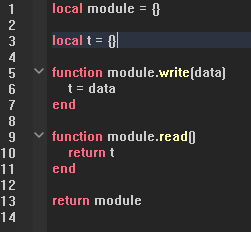# I can't write data inside of an script and read it out

I have made a data store system, and then I encountered a problem that I can’t use the data I have loaded from the server.

I made a module script so that I can deal with it easier, I found out that the “t” table only changes when it’s inside of the “Write” function. So, after the write function is called. The read function read nothing from the t table.

I can’t really come up with a valid solution, I tried searching.Maybe you are using require every time when you run

``````local module = {}

local t = {}

function module.write(data)
t = data
end

return t
end

module.write({"test"})

for index, value in next, t do
print(index, value)
end

--[[
Output:
1	test
]]
``````

Why do you need to do this process? you just do something like this:

``````local module = {}

function module:new()
local self = {}

return self
end

return module
``````

You can read the content and write at the same time. Also if you really want this approach you will need a key.

``````local module = {}

local t = {}

function module.write(key, value)
if typeof(key) == "string" then
t[key] = value
elseif typeof(key) == "number" then
table.insert(t, value)
else
warn("Invalid key!")
end
end

return t
end

return module
``````

I loaded the whole data when the player joins the game, the main script and this one are on the clientside. So, this script is work as a storage in the client side.

If so then you can just use this:

Since this works the same way this is local sided:

``````local module = require(...)
local newData = module:new()

newData["test"] = "test"

print(newData)
--[[
["test"] = "test"
]]
``````

The one that you made is not since that would update everytime you call the `module.write` if its in the server.

So, this works the same as the write function?

1 Like

Yes this is called a class in other programming language, in lua’s case it uses table as class, lua’s table can be everything that other programming language does not list, tuple, dictionary, even `math` is a table `math.sin, math.floor, etc.`.

Still, nothing has written in.

The first time ‘require’ is called on a ModuleScript, its contents are executed and the value it returns is returned by the `require` function, this returned value is cached. If the same ModuleScript is further required, instead of its contents being re-executed the cached value is simply returned by the `require` function. ModuleScripts that return a table value return a reference to that table value (as tables are immutable).